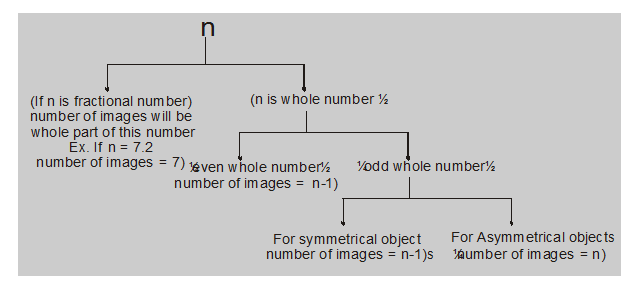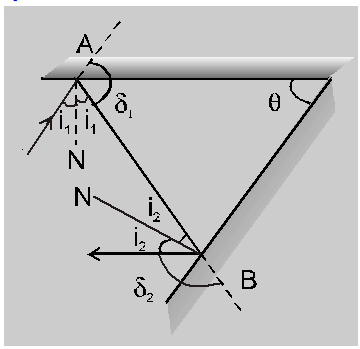Most Affordable JEE | NEET | 8,9,10 Preparation by Kota's Top IITian Doctor Faculties

# Reflection by plane mirror - Ray Optics, Physics - eSaral`

Hey, do you want to learn about the Reflection by a plane mirror? If yes. Then keep reading

## Reflection by plane mirror:

1. The image formed by the plane mirror is always erect, of the same size, and at the same distance as the object is.
2. To see the full image in a plane mirror, its length is just half the height of the man and it has to be kept in a specific position.
3. When the plane mirror or any reflecting surface is turned through an angle $\theta$ and if the incident ray remains stationary, then the reflected ray will turn through $2 \theta$.
4. The image of an object formed by a plane mirror is perverted & if the object is real then a virtual image formed.
5. Image due to a plane mirror is as far behind the mirror as is the object in Infront of it.
6. The magnification produced by the plane mirror is 1 i.e. the size of the image is equal to the size of the object.
7. When the two plane mirrors are parallel to each other, the number of images is infinity.
8. When two plane mirrors is held at angle $\theta$ with their reflecting surfaces facing each other and an object is placed between them, images are formed by successive reflections.

First of all, we will calculate

$n=\frac{360}{\theta}$

Then9. If the angle between the two mirrors is $\theta$, the deviation produced by successive reflections is

$\delta=\delta_{1}+\delta_{2}=2 \pi-2 \theta$Note : When reflection takes place by a smooth surface, it is called regular reflection, but when reflection takes place by a rough surface, it is called diffused reflection.

So, that's all from this article. I hope you get the idea about Reflection by a plane mirror. If you found this article informative then please share it with your friends. If you have any confusion related to this topic then feel free to ask in the comments section down below.

For a better understanding of this chapter, please check the detailed notes of Ray Optics. To watch Free Learning Videos on physics by Saransh Gupta sir Install the eSaral App.